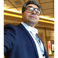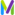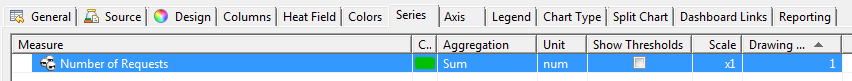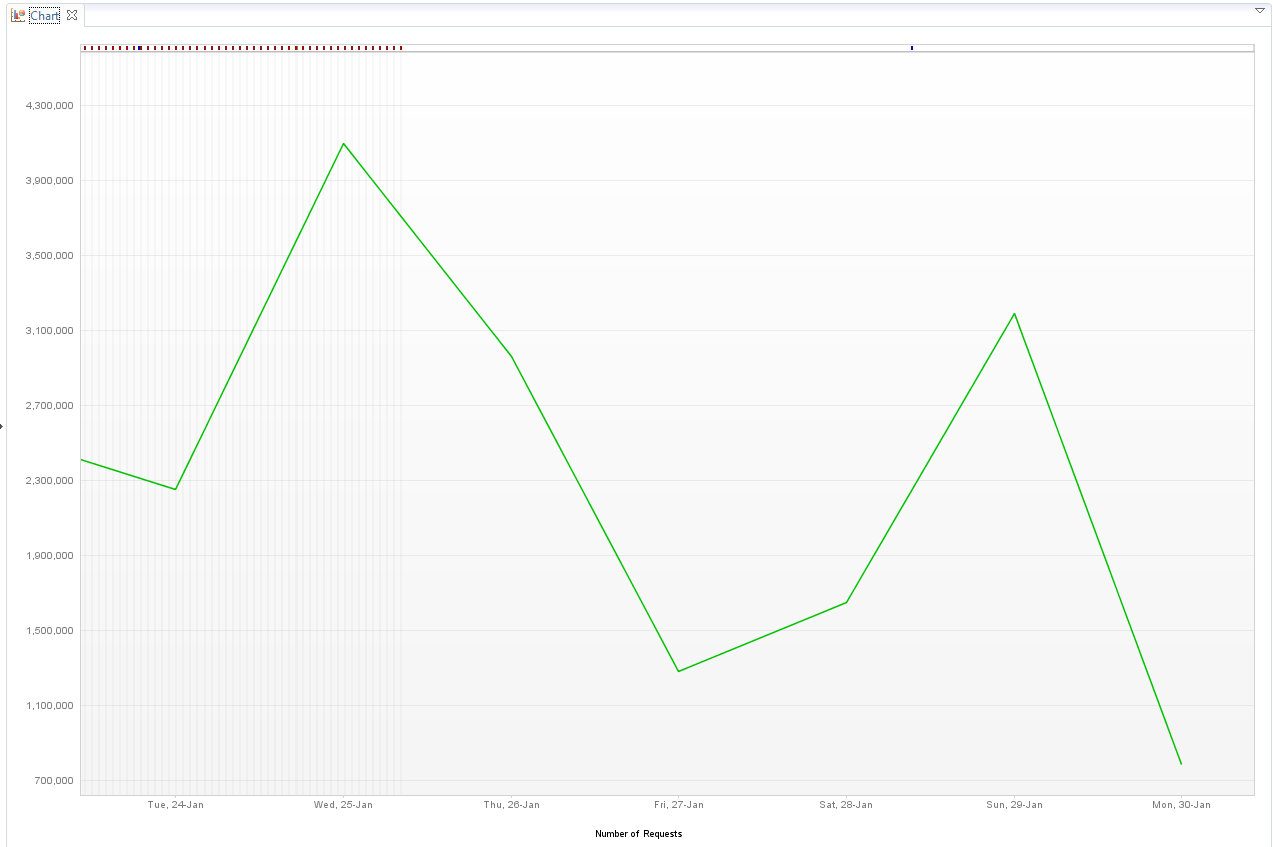cancel
Showing results for
Show  only  | Search instead for
Did you mean:

This product reached the end of support date on March 31, 2021.

## how could I check the transactions amount of each days?Inactive

This is Ryotaro.

I want to use the Sizing Calculator tool for new dynaTrace6.3 environment,
However, I'm not sure How should I know "How high is the max. peak factor"?

(We have the environment that working dynaTrace 5.6.)

Could you tell me how could I check the transactions amount of each days?Dear Daichi,

Below is the criteria to configure the load.

#### Collect PurePath Numbers

Estimate the number of transactions (this is the number of server-side requests) that are handled by the data center during one day. Normally, operators have a good understanding of how many transactions are produced. If you don't have this number, you can also estimate it with a POC in which you can see the current transactions per seconds in the Client, and then compute the daily transactions.

In addition, you may need to estimate the number of nodes per PurePath (or transaction). The number of nodes may vary. In some environments, the number is relatively low (about 50), but our experience leads us to recommend higher estimates (200 to 300). You can empirically derive the number of nodes with self-monitoring during a POC.

#### UEM Considerations

If you use UEM, review the static resources.

Are static resources delivered by the web server? If yes, this leads to a higher number of transactions with only one node. A typical web request contains some 40 static resources, 80 percent of which are cached. As a result, the number of transactions per second is increased, but the average number of nodes per PurePath is lowered due to the caching. Within a UEM environment, the average Nodes per PurePath are estimated with the following formula:

(Transactions per Day * average Nodes per PurePath + Static Resources requested per Day) / (Transactions per Day + Static Resources requested per Day).

Add a dashlet with the measure of number of requests, change the timeframe last 7 days and get the aggregation as daily (second screenshot as a reference).Regards,

Babar